# Samacheer Kalvi 7th Maths Solutions Term 2 Chapter 3 Algebra Ex 3.1

Students can Download Maths Chapter 3 Algebra Ex 3.1 Questions and Answers, Notes Pdf, Samacheer Kalvi 7th Maths Book Solutions Guide Pdf helps you to revise the complete Tamilnadu State Board New Syllabus and score more marks in your examinations.

## Tamilnadu Samacheer Kalvi 7th Maths Solutions Term 2 Chapter 3 Algebra Ex 3.1

Question 1.
Fill in the blanks.

1. The exponential form 149 should be read as ______
2. The expanded form of p3 q2 is ______
3. When base is 12 and exponent is 17, its e×ponential form is _____
4. The value of (14 × 21)0 is _____

1. 14 Power 9
2. p × p × p × q × q
3. 1217
4. 1

Question 2.
Say True or False.

1. 23 × 32 = 65
2. 29 × 32 = (2 × 3)9×2
3. 34 × 37= 311
4. 20 × 10000
5. 23 < 32

1. False
2. False
3. True
4. True
5. TrueQuestion 3.
Find the value of the following.

1. 26
2. 112
3. 54
4. 93

Solution:

1. 26 = 2 × 2 × 2 × 2 × 2 × 2 = 64
2. 112 = 11 × 11 = 121
3. 54 = 5 × 5 × 5 × 5 = 625
4. 93 = 9 × 9 × 9 = 729

Question 4.
Express the following in e×ponential form.

1. 6 × 6 × 6 × 6
2. t × t
3. 5 × 5 × 7 × 7 × 7
4. 2 × 2 × a × a

Solution:

1. 6 × 6 × 6 × 6 = 61+1+1+1 = 64 [Since am × an = am+n]
2. t × t = t1+1 = t2
3. 5 × 5 × 7 × 7 × 7 = 51+1 × 71+1+1 = 52 × 73
4. 2 × 2 × a × a = 21+1 × a1+1 = 22 × a2 = (2a)2Question 5.
E×press each of the following numbers using e×ponential form,
(i) 512
(ii) 343
(iii) 729
(iv) 3125
Solution:
(i) 512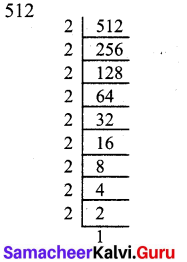512 = 2 × 2 × 2 × 2 × 2 × 2 × 2 × 2 × 2 × 2
= 29 [Using product rule]

(ii) 343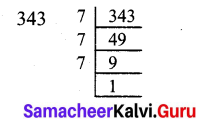343 = 7 × 7 × 7 = 71+1+1
= 73 [Using product rule]

(iii) 729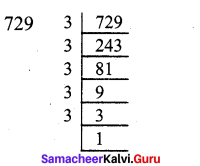729 = 3 × 3 × 3 × 3 × 3 × 3
= 36 [Using product rule]

(iv) 3125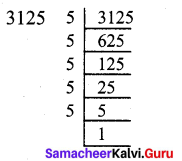3125 = 5 × 5 × 5 × 5 × 5
= 55 [Using product rule]Question 6.
Identify the greater number in each of the following.
(i) 63 or 36
(ii) 53 or 35
(iii) 28 or 82
Solution:
(i) 63 or 36
63 = 6 × 6 × 6 = 36 × 6 = 216
36 = 3 × 3 × 3 × 3 × 3 × 3 = 729
729 > 216 gives 36 > 63
∴ 36 is greater.

(ii) 53 or 35
53 = 5 × 5 × 5 = 125
35 = 3 × 3 × 3 × 3 × 3 = 243
243 > 125 gives 35 > 53
∴ 35 is greater.

(iii) 28 or 82
28 = 2 × 2 × 2 × 2 × 2 × 2 × 2 × 2 = 256
82 = 8 × 8 = 64
256 > 64 gives 28 > 82
∴ 28 is greater.Question 7.
Simplify the following
(i) 72 × 34
(ii) 32 × 24
(iii) 52 × 104
Solution:
(i) 72 × 34 = (7 × 7) × (3 × 3 × 3 × 3)
= 49 × 81 = 3969

(ii) 32 × 24 = (3 × 3) × (2 × 2 × 2 × 2)
= 9 × 16 = 144

(iii) 52 × 104 = (5 × 5) × (10 × 10 × 10 × 10)
= 25 × 10000 = 2,50,000

Question 8.
Find the value of the following.
(i) (-4)2
(ii) (-3) × (-2)3
(iii) (-2)3 × (-10)3
Solution:
(i) (-4)2 = (-1)2 × (4)2 [since am × bm = (a × b)m]
= 1 × 16 = 16 [since (-1)n = 1 if n is even]

(ii) (-3) × (-2)3 = (-1) × (-3) × (-1)3 × (-2)3
= (-1)4 × 24 [Grouping the terms of same base]
= 24

(iii) (-2)3 × (-10)3 = (-1)3 × (-2)3 × (-1)3 × (-10)3
= (-1)3+3 × 23 × 103 [Grouping the terms of same base]
= (-1)6 × (2 × 10)3
[∵ am × bm = (a × b)m]
= 1 × 203 [since (-1)n = 1 if n is even]
= 8000Question 9.
Simplify using laws of exponents.
(i) 35 × 38
(ii) a4 × a10
(iii) 7x × 72
(iv) 25 ÷ 23
(v) 188 ÷ 184
(vi) (64)3
(vii) (xm)0
(viii) 95 × 35
(ix) 3y × 12y
(x) 256 × 56
Solution: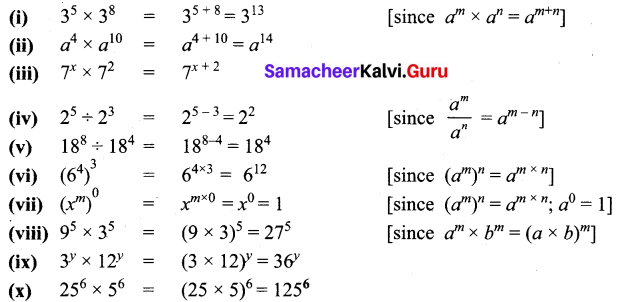Question 10.
If a = 3 and b = 2, then find the value of the following.
(i) ab + ba
(ii) aa – bb
(iii) (a + b)b
(iv) (a – b)a
Solution:
(i) ab + ba
a = 3 and b = 2
we get 32 + 23 = (3 × 3) + (2 × 2 × 2) = 9 + 8 = 17

(ii) (aa – bb)
Substituting a = 3 and b = 2
we get 32 – 22 = (3 × 3 × 3) – (2 × 2) = 27 – 4 = 23

(iii) (a + b)b
Substituting a = 3 and b = 2
we get (3 + 2)2 = 52 = 5 × 5 = 25

(iv) (a – b)a
Substituting a = 3 and b = 2
we get (3 – 2)3 = 13 = 1 × 1 × 1 = 1Question 11.
Simplify and express each of the following in exponential form:
(i) 45 × 42 × 44
(ii) (32 × 33)7
(iii) (52 × 58) ÷ 5s
(iv) 20 × 30 × 40
(v) $$\frac{5^{5} \times a^{8} \times b^{3}}{4^{3} \times a^{5} \times b^{2}}$$
Solution: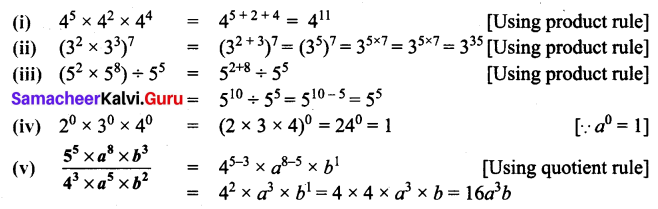Objective Type Questions

Question 12.
a × a × a × a × a equal to
(i) a5
(ii) 5 a
(iii) 5a
(iv) a + 5
(i) a5

Question 13.
The exponential form of 72 is
(i) 72
(ii) 27
(iii) 22 × 33
(iv) 23 × 32
(iv) 23 × 32

Question 14.
The value of x in the equation a13 = x3 × a10 is
(i) a
(ii) 13
(iii) 3
(iv) 10
(i) a

Question 15.
How many zeros are there in 10010 ?
(i) 2
(ii) 3
(iii) 100
(iv) 20